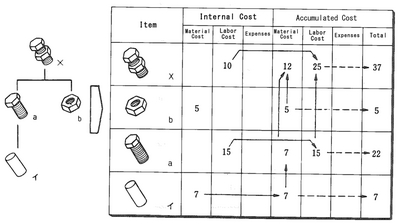#### Asprova MRP

Asprova MRP is calculated on the memory requirement, allocate lead time at a high speed. Can achieve material arrangement Just in Time, which is impossible in other MRP system.

MRP glossary TOP > Cost Control > Accumulated Cost

## Accumulated Cost

Focusing on a certain item, the value obtained by adding the external cost (the cost of assembling or manufacturing constituent items) to the internal cost of the item itself is called Accumulated Cost. For example, the internal cost of Product X is the labor cost (10 yen), and the external cost of Product X is the processing cost of Part a (15 yen) and the material cost of Part b and material "イ" (12 yen). In this case, the accumulated costs of Product X is as follows: material cost: 12 yen, labor cost: 25 yen, and product cost: 37 yen.In this way, the accumulated cost of each item is calculated by piling up internal costs of items such as assemblies, parts, and raw materials, which is generally called Standard Costing by Product or Accumulating Costs.
In order to calculate this accumulated cost by computer, the fields of internal cost and accumulated cost as the cost data should be set for items in B/M. Each field has the fields of material cost, labor cost, and expenses as specifications. In case of factories which have many subcontract-related items, the field of subcontract processing cost is also set. The field of internal cost may be divided into standard cost and current standard cost with its cost elements. In every case, the accumulated cost is used for making a cost estimate, changing cost, standard cost accounting.

Related term: Internal Cost and External Cost

Reference：JIT Business Research Mr. Hirano Hiroyuki